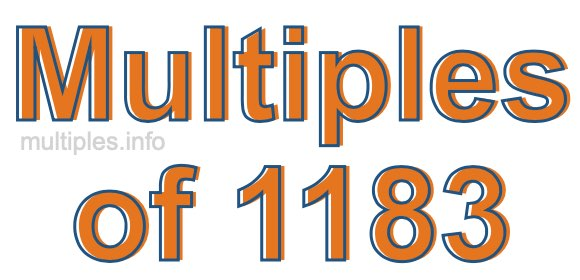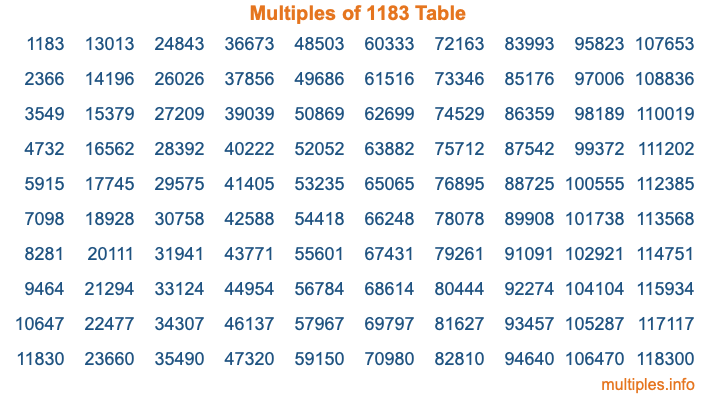Multiples of 1183Welcome to the Multiples of 1183 page. Here we will first teach you everything you will ever need to know about the multiples of 1183, and then give you a study guide summary of everything we taught you to make sure you remember it all. Use this page to look up facts and learn information about the multiples of 1183. This page will make you a multiples of one thousand one hundred eighty-three expert!

Definition of Multiples of 1183
Multiples of 1183 are all the numbers that when divided by 1183 equal an integer. Each of the multiples of 1183 are called a multiple. A multiple of 1183 is created by multiplying 1183 by an integer.

Therefore, to create a list of multiples of 1183, you start with 1 multiplied by 1183, then 2 multiplied by 1183, then 3 multiplied by 1183, and so on for as long as you want. Thus, the list of the first five multiples of 1183 is 1183, 2366, 3549, 4732, and 5915. To see a larger list of multiples of 1183, see the printable image of Multiples of 1183 further down on this page. We also have a category where you can choose any nth multiple of 1183.

Multiples of 1183 Checker
The Multiples of 1183 Checker below checks to see if any number of your choice is a multiple of 1183. In other words, it checks to see if there is any number (integer) that when multiplied by 1183 will equal your number. To do that, we divide your number by 1183. If the the quotient is an integer, then your number is a multiple of 1183.

Is  a multiple of 1183?

Least Common Multiple of 1183 and ...
A Least Common Multiple (LCM) is the lowest multiple that two or more numbers have in common. This is also called the smallest common multiple or lowest common multiple and is useful to know when you are adding our subtracting fractions. Enter one or more numbers below (1183 is already entered) to find the LCM.

Check out our LCM Calculator if you need more details about the Least Common Multiple or if you need the LCM for different numbers for adding and subtraction fractions.

nth Multiple of 1183
As we stated above, 1183 is the first multiple of 1183, 2366 is the second multiple of 1183, 3549 is the third multiple of 1183, and so on. Enter a number below to find the nth multiple of 1183.

th multiple of 1183

Multiples of 1183 vs Factors of 1183
1183 is a multiple of 1183 and a factor of 1183, but that is where the similarities end. All postive multiples of 1183 are 1183 or greater than 1183. All positive factors of 1183 are 1183 or less than 1183.

Below is the beginning list of multiples of 1183 and the factors of 1183 so you can compare:

Multiples of 1183: 1183, 2366, 3549, 4732, 5915, etc.

Factors of 1183: 1, 7, 13, 91, 169, 1183

As you can see, the multiples of 1183 are all the numbers that you can divide by 1183 to get a whole number. The factors of 1183, on the other hand, are all the whole numbers that you can multiply by another whole number to get 1183.

It's also interesting to note that if a number (x) is a factor of 1183, then 1183 will also be a multiple of that number (x).

Multiples of 1183 vs Divisors of 1183
The divisors of 1183 are all the integers that 1183 can be divided by evenly. Below is a list of the divisors of 1183.

Divisors of 1183: 1, 7, 13, 91, 169, 1183

The interesting thing to note here is that if you take any multiple of 1183 and divide it by a divisor of 1183, you will see that the quotient is an integer.

Multiples of 1183 Table
Below is an image of the first 100 multiples of 1183 in a table. The table is in chronological order, column by column. The first column has the first ten multiples of 1183, the second column has the next ten multiples of 1183, and so on.The Multiples of 1183 Table is also referred to as the 1183 Times Table or Times Table of 1183. You are welcome to print out our table for your studies.

Negative Multiples of 1183
Although not often discussed or needed in math, it is worth mentioning that you can make a list of negative multiples of 1183 by multiplying 1183 by -1, then by -2, then by -3, and so on, to get the following list of negative multiples of 1183:

-1183, -2366, -3549, -4732, -5915, etc.

Multiples of 1183 Summary
Below is a summary of important Multiples of 1183 facts that we have discussed on this page. To retain the knowledge on this page, we recommend that you read through the summary and explain to yourself or a study partner why they hold true.

There are an infinite number of multiples of 1183.

A multiple of 1183 divided by 1183 will equal a whole number.

1183 divided by a factor of 1183 equals a divisor of 1183.

The nth multiple of 1183 is n times 1183.

The largest factor of 1183 is equal to the first positive multiple of 1183.

1183 is a multiple of every factor of 1183.

1183 is a multiple of 1183.

A multiple of 1183 divided by a divisor of 1183 equals an integer.

1183 divided by a divisor of 1183 equals a factor of 1183.

Any integer times 1183 will equal a multiple of 1183.

Multiples of a Number
Here you can get the multiples of another number, all with the same attention to detail as we did for multiples of 1183 on this page.

Multiples of
Multiples of 1184
Did you find our page about multiples of one thousand one hundred eighty-three educational? Do you want more knowledge? Check out the multiples of the next number on our list!